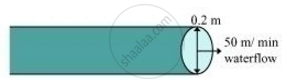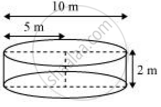Share

# A farmer connects a pipe of internal diameter 20 cm form a canal into a cylindrical tank in her field, which is 10 m in diameter and 2 m deep. If water flows through the pipe at the rate of 3 km/h, in how much time will the tank be filled? - CBSE Class 10 - Mathematics

ConceptConversion of Solid from One Shape to Another

#### Question

A farmer connects a pipe of internal diameter 20 cm form a canal into a cylindrical tank in her field, which is 10 m in diameter and 2 m deep. If water flows through the pipe at the rate of 3 km/h, in how much time will the tank be filled?

#### SolutionConsider an area of cross-section of pipe as shown in the figure.

Radius (r1) of circular end of pipe = 20/200 = 0.1 m

Area of cross-section = pixxr_1^2 = pixx(0.1)^2 = 0.01pi m^2

Speed of water = 3 km/h = 3000/60 = 50 meter/min

Volume of water that flows in 1 minute from pipe = 50 × 0.01 π = 0.5π m3

Volume of water that flows in t minutes from pipe = t × 0.5π m3Radius (r2) of circular end of cylindrical tank = 10/2 = 5m

Depth (h2) of cylindrical tank = 2 m

Let the tank be filled completely in t minutes.

Volume of water filled in tank in t minutes is equal to the volume of water flowed in t minutes from the pipe.

Volume of water that flows in t minutes from pipe = Volume of water in tank

t × 0.5π = π ×(r2)2 ×h2

t × 0.5 = 52 ×2

t = 100

Therefore, the cylindrical tank will be filled in 100 minutes.

Is there an error in this question or solution?

#### APPEARS IN

NCERT Solution for Mathematics Textbook for Class 10 (2019 to Current)
Chapter 13: Surface Areas and Volumes
Ex. 13.30 | Q: 9 | Page no. 252

#### Video TutorialsVIEW ALL 

Solution A farmer connects a pipe of internal diameter 20 cm form a canal into a cylindrical tank in her field, which is 10 m in diameter and 2 m deep. If water flows through the pipe at the rate of 3 km/h, in how much time will the tank be filled? Concept: Conversion of Solid from One Shape to Another.
S